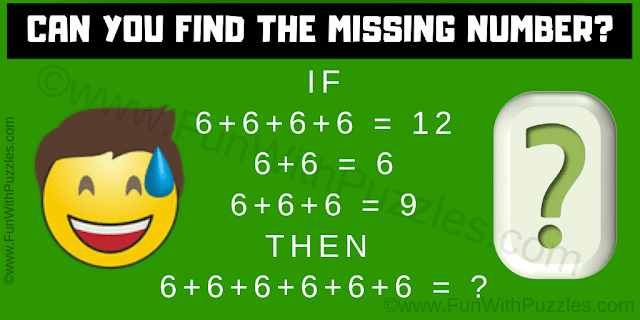## Wednesday, June 26, 2019

This is an easy and quick maths logic question for kids. This is an easy puzzle question, so try to answer this as quickly as possible. In this math logic puzzle, you are shown some maths equations. Find the logical sequence or pattern used in these equations and then find the value of the missing number which will be the answer to the last number equation.Can you find the missing number?
Answer of this "Logic Math Question", can be viewed by clicking on the answer button.